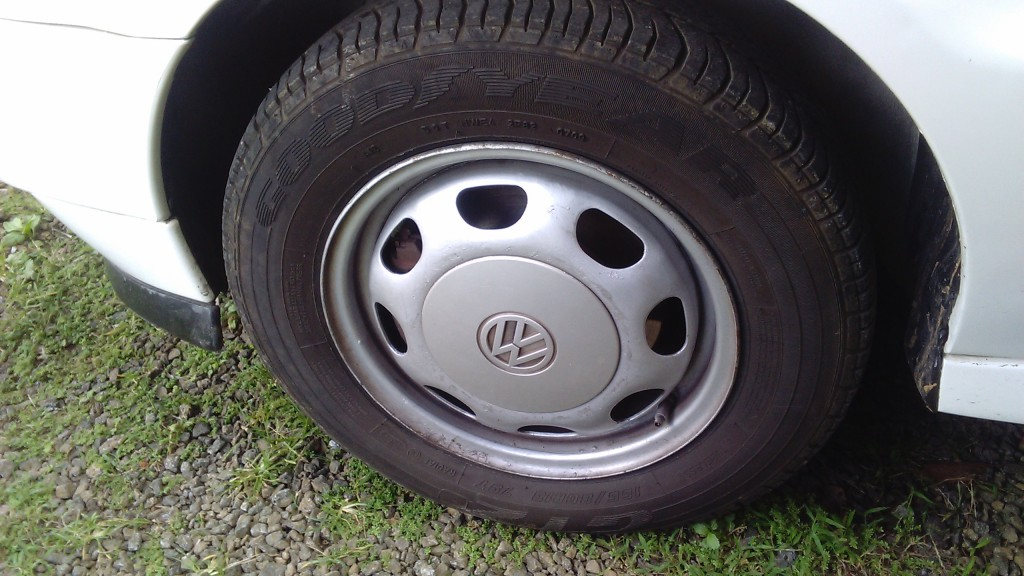# Changing a Car Tyre

Dad noticed my car front tyres were different in size. Maybe one was flat or whatever? No, they were indeed of different size. Here’s there specs:

Front left  : 155/80 R13

Front right: 155/70 R13

As you can see the aspect ratio of the tyres are different. Here’s a diagram below explaining the tyre and wheels terminologies:

Let’s calculate the circumference of the 2 tyres

$$\text{tyre width}_{t1} = \frac{155mm}{1000} = 0.155m$$

$$\text{rim diameter}_{t1} = 13\text{inch} \times 0.0254 = 0.3302 m$$

$$\text{rim radius}_{t1} = \frac{0.3302m}{2} = 0.1651m$$

$$\text{tyre height}_{t1} = \text{tyre width} \times \text{aspect ratio} = 0.155m \times 80\% = 0.124m$$

$$\text{tire radius}_{t1} = \text{rim radius} + \text{tyre height} = 0.1651m + 0.124m = 0.2891$$

$$\text{tyre circumference} _{t1}= 2 \times \pi \times \text{tyre radius} = 2 \times \pi \times 0.2891 = 1.816m$$

Lemme try to write a function for getting the tyre diameter with variables w as tyre width, a as aspect ratio and r as rim diameter.

$$f(w,a,r) = 2 \times \pi \times (\frac{r \times 0.0254}{2} + \frac{w \times 80}{1000 \times 100})$$

Let’s calculate the diameter of the second tyre:

$$\text{tyre circumference}_{t2} = f(155,70,13) = 1.719m$$

The difference in diameter is about $$0.097m \text{ or } 9.7cm$$

Does it matter if the 2 tyres are of different sizes?

Most cars in Mauritius are front-wheel-drive (FWD) cars. My VW Polo MK3 is too. Distinction is made between Front-wheel-drive rear-wheel-drive (RWD) cars by which wheels are connected to the engine; the drivetrain to be more precise.

In RWD cars:
– The front tyres handle the steering part.
– The rear tyres are responsible to acceleration the vehicle.
– Braking is divided among front and rear tyres.

In FWD cars, all the roles are performed by the front-tyres only except for braking which both rear and front do. I am not going to discuss FWD vs RWD in this blog post.

Ok, I was situation that my 2 powered tyres happened to be of different size. The 2 wheels are designed in such a way that they do not spin in strict synchrony with each other. The reason is simple, imagine you are going through a corner, your inner tyres will need to cover less distance than the outer tyre. Remember our circumference equation above? If the wheels were made to rotate at the same speed, the inner wheel would slip causing loss of stability. Car’s are fitted with a differential to allow wheels to spin at different speeds while be able to power them. The disadvantage of this is that most power goes to the wheel with the least friction. If one of your drive tyre is in the air, the other drive tyre will remain still and the tyre in air will spin leaving you puzzled why the car is not moving. To solve this problem, engineers invented the limited slip differential. Think I’m going into too much details. Back to topic:

If the 2 FWD tyres are different, it shall not cause tyres to slip due to presence of an Open Differential. But the smaller tyre would be the one accelerating your car since it requires less torque to get spinning. Thus, only 1 wheel is responsible for power delivery on a total of 4 wheels. That’s kinda unfair. It is also going to have much more wear than the others. This can cause the car to tend to either left or right as i was experiencing.Do make sure that the 2 tyres connected to the drivetrain are of the same size!

To upscale or downscale?
Ok, we got a bigger tyre and a smaller tyre. Do we replace the bigger one with a new smaller one or vice versa?

First advice, have a look at your owners manual. It will have best tyre size depending on your engine size.

Putting a bigger tyre than stock might give your car better looks and top speed but you lose in terms of acceleration and also fuel economy (rumour says).

Maintaining a car needs lots of time, money and research. Take care of your car as much as you can 🙂